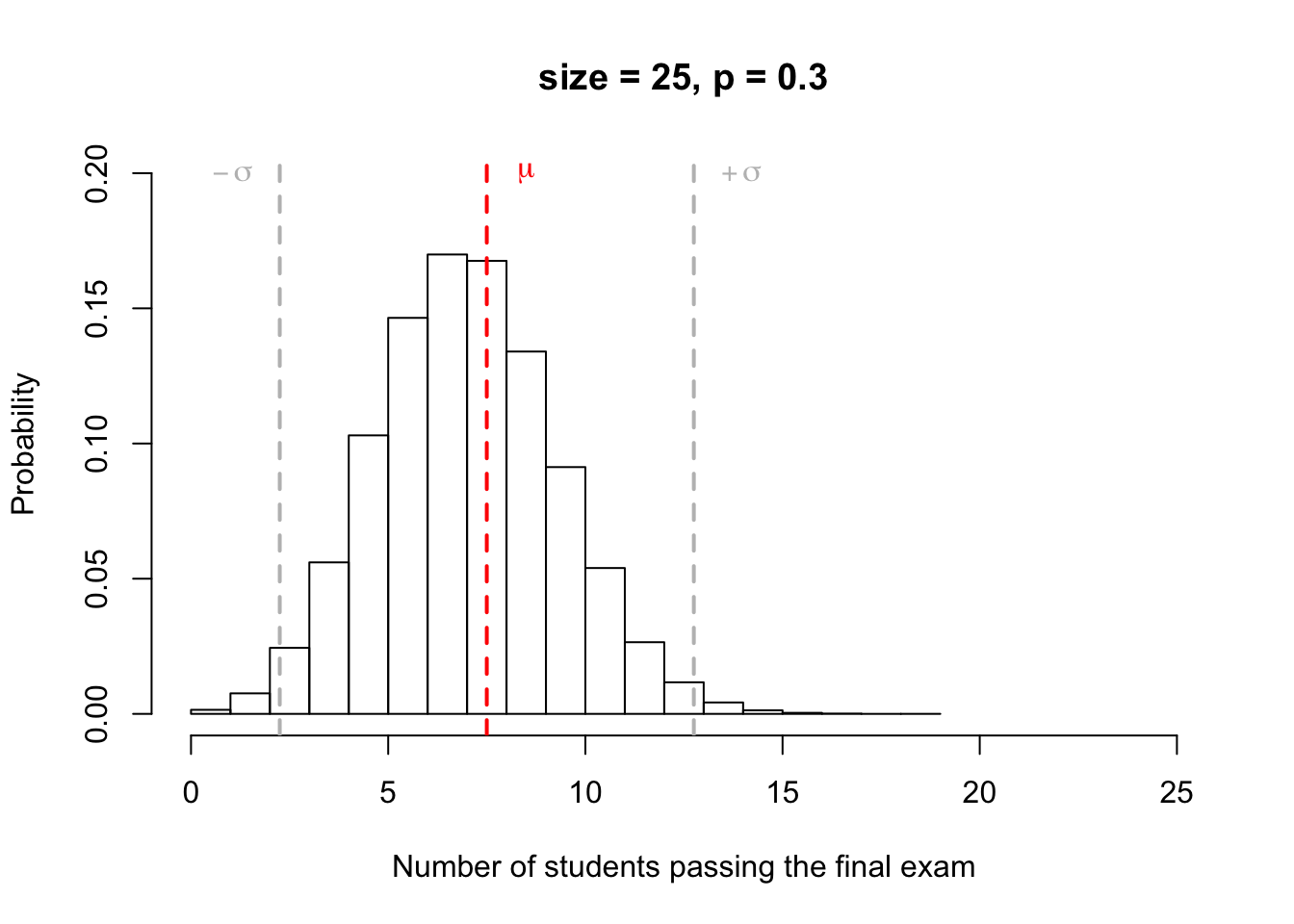The mean and standard deviation of a binomial random variable with parameters $$n$$ and $$p$$ are

$\mu = np$ and $\sigma = np(1 - p)\text{,}$ respectively.

Let us recall the example, from the previous section. The probability to pass the final statistic exam is 0.3. We consider a class of 25 students. The random variable $$X$$, which corresponds to success in the exam is a binomial random variable, and follows a binomial distribution with parameters $$n=25$$ and $$p=0.3$$. Thus the mean, $$\mu$$, and the standard deviation, $$\sigma$$, can be computed as follows:

$\mu = np = 25 \times 0.3 = 7.5$

and

$\sigma = np(1 - p) = 25 \times 0.3 \times (1-0.3) = 5.25$

The following plot visualizes the binomial distribution with parameters $$n=25$$ and $$p=0.3$$, and its mean $$\mu$$ and standard deviation $$\sigma$$.